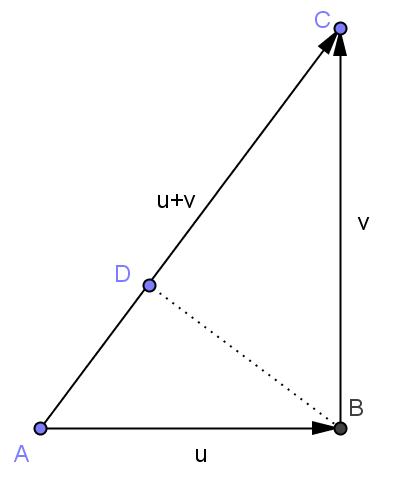# Linear Algebra Done Right第二章注记和部分习题

1 线性无关和子空间直和的联系

1）二者的概念同源，都是要将一个大的空间的向量唯一地分解成若干小的子空间中向量的和。
2）v1,v2,…,vn线性无关的充要条件是v1,v2,…,vn都不等于0且span(v1),span(v2),…,span(vn)的和是直和。
3）设有若干组向量：向量组u1,u2,…,ur，向量组v1,v2,…,vs，和向量组w1,w2,…,wt，每一组向量都线性无关，把它们放在一起还是线性无关吗？这显然是不一定的。但是怎样才能保证把它们放在一起也线性无关呢？有以下两个结果：
a）向量组u1,u2,…,ur，向量组v1,v2,…,vs，和向量组w1,w2,…,wt，每一组向量都线性无关，则向量组u1,…,ur,v1,…,vs,w1,…,wt线性无关当且仅当span(u1,u2,…,ur)+span(v1,v2,…,vs)+span(w1,w2,…,wt)是直和。
b）若U1+U2+…+Un是直和，那么分别从U1,U2,…,Un每个子空间中任意选出一组线性无关的向量，它们整体还是线性无关的。

2 33页定理2.18（dim(U1 + U2) = dimU1 + dimU2 − dim(U1 ∩ U2)）的证明思路问题

a1u1 + ··· + amum + b1v1 + ··· + bjvj + c1w1 +· · ·+ckwk = 0

3 维数定理和有限集合基数定理（容斥原理）

（略）

# Linear Algebra Done Right第一章注记和部分习题

1）$$V_1+V_2$$是直和；
2）$$V_1 \cap V_2=\{0\}$$

9 证明$$V$$的两个子空间的并集也是$$V$$的子空间当且仅当其中一个子空间包含于另一个子空间。

# 用向量的内积证明勾股定理——体会代数的威力

||u+v||²=<u+v,u+v>=<u,u+v>+<v,u+v>=<u,u>+<u,v>+<v,u>+<v,v>。4月27日有关余弦定理的补充：

||u-v||²=<u-v,u-v>=<u,u>+<v,v>-<u,v>-<v,u>=||u||²+||v||²-2<u,v>
||u+v||²=<u+v,u+v>=<u,u>+<v,v>+<u,v>+<v,u>=||u||²+||v||²+2<u,v>

<u,v>=<v,u>=0，和勾股定理||u-v||²=||u+v||²=||u||²+||v||²

# 向量外积与行列式的本质关系

1) |a×b|=|a||b|sin<a,b> 其中sin<a,b>表示a和b两向量夹角的正弦。
2) 若|a×b|≠0，那么a×b的方向垂直于a和b所在平面并且使a,b,c满足右手螺旋定则。

1) a×b = -b×a
2) (a+b)×c = a×c+b×c
3) a×(b+c) = a×b+a×c
4) a×(kb)=(ka)×b=k(a×b)
5) i×j=k,j×k=i,k×i=j

$$\displaystyle \textbf{a}\times \textbf{b}=(a_1i+a_2j+a_3k)\times (b_1i+b_2j+b_3k)$$

$$\displaystyle \textbf{c}=( \begin{vmatrix}a_2&a_3\\b_2&b_3\end{vmatrix},-\begin{vmatrix}a_1&a_3\\b_1&b_3\end{vmatrix},\begin{vmatrix}a_1&a_2\\b_1&b_2\end{vmatrix})$$

\begin{aligned}\textbf{c}\cdot\textbf{a}&=a_1\begin{vmatrix}a_2&a_3\\ b_2&b_3\end{vmatrix}-a_2\begin{vmatrix}a_1&a_3\\ b_1&b_3\end{vmatrix}+a_3\begin{vmatrix}a_1&a_2\\ b_1&b_2\end{vmatrix}\\ &=\begin{vmatrix} a_1&a_2&a_3\\ a_1&a_2 &a_3\\ b_1&b_2&b_3\end{vmatrix}\\ &=0\end{aligned}

\begin{aligned}\textbf{c}\cdot\textbf{b}&=b_1\begin{vmatrix}a_2&a_3\\ b_2&b_3\end{vmatrix}-b_2\begin{vmatrix}a_1&a_3\\ b_1&b_3\end{vmatrix}+b_3\begin{vmatrix}a_1&a_2\\ b_1&b_2\end{vmatrix}\\ &=\begin{vmatrix} b_1&b_2&b_3\\ a_1&a_2 &a_3\\ b_1&b_2&b_3\end{vmatrix}\\ &=0\end{aligned}

| a1 a2 a3 |
| b1 b2 b3 |
| c1 c2 c3 |

| a1 a2 a3 |
| b1 b2 b3 |
| c1 c2 c3 |

|c| ² =|a| |b| sin<a,b> |c| (因为体积等于底面积乘以高)

| a1 a2 a3 |
| b1 b2 b3 |
| d1 d2 d3 |

a1,a2, …,an-1,a1×a2×…an-1取正向，那么必须在表示外积的行列式中把基底那一行写在最下面，然后按照基底那一行展开成各个分量。

1）行列式，2）3个向量张成多面体的有向体积，3）2个向量的外积，4）3个向量的混合积